•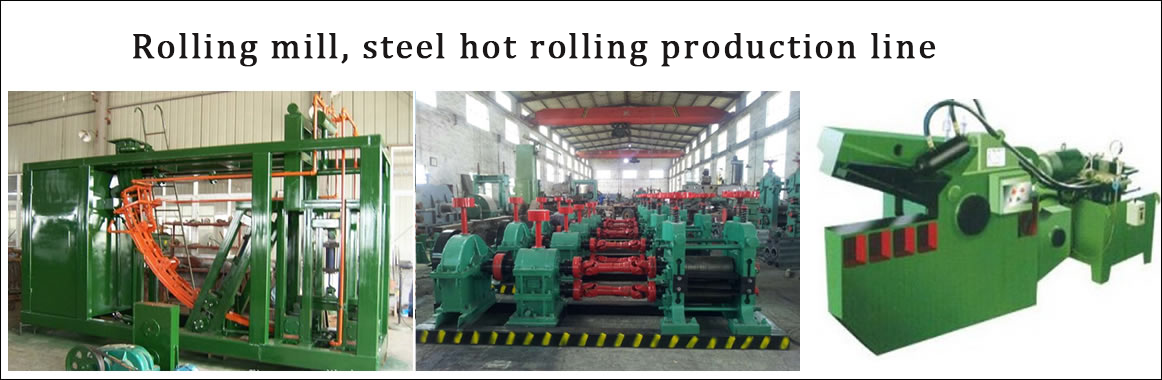•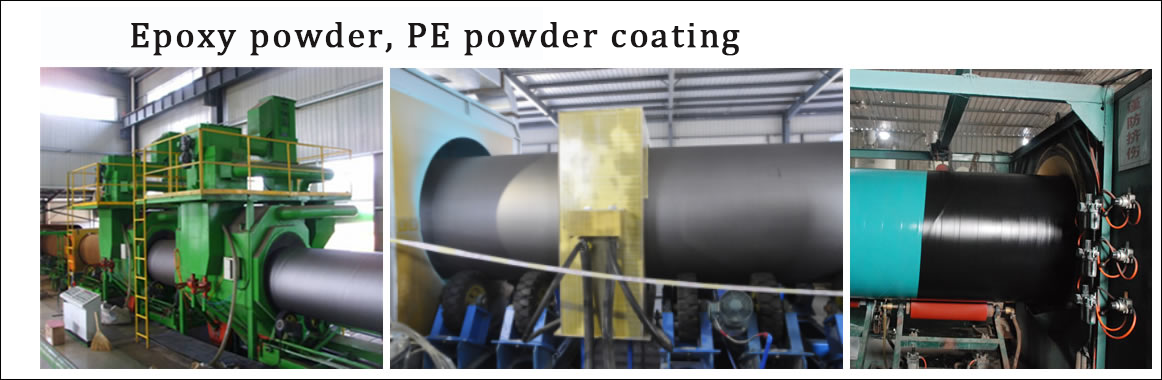•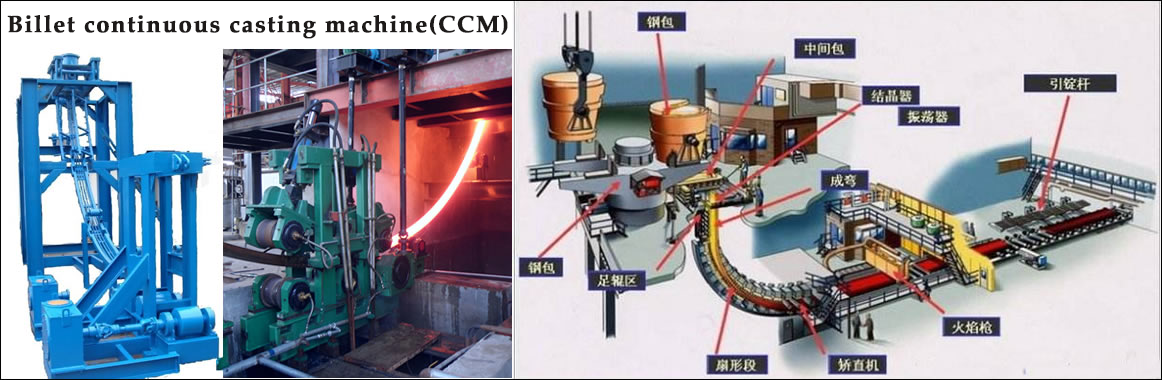•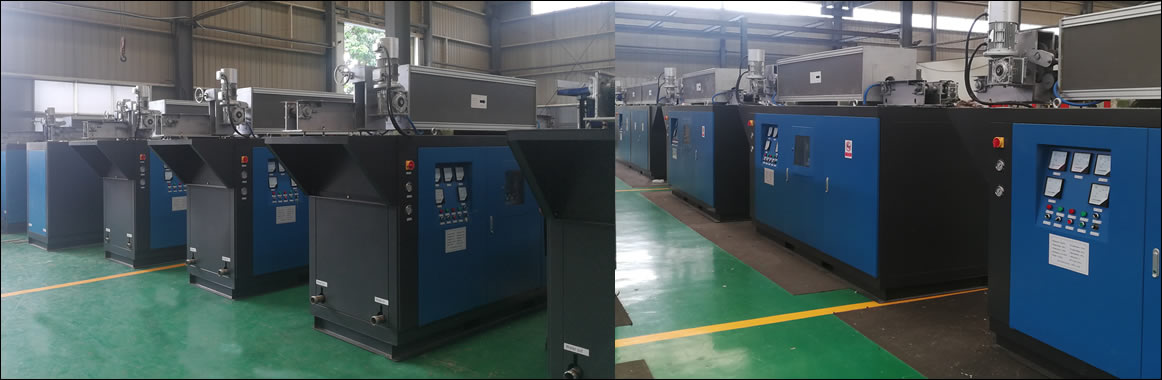•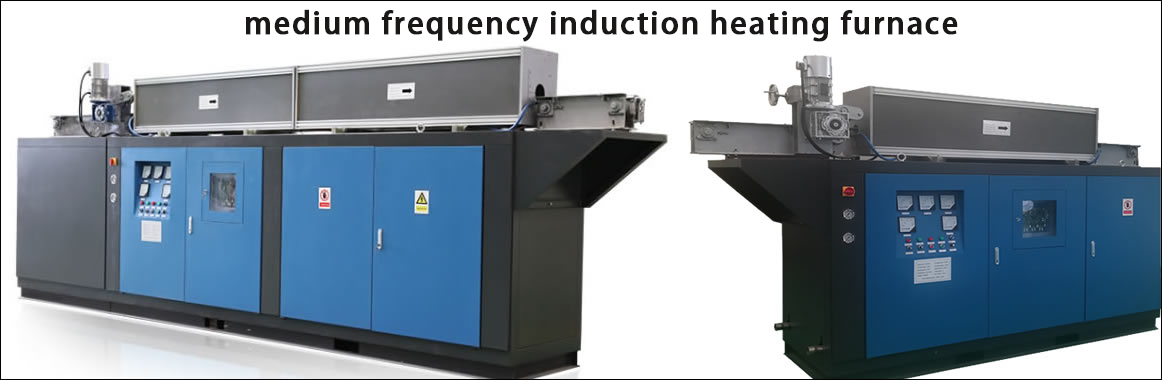•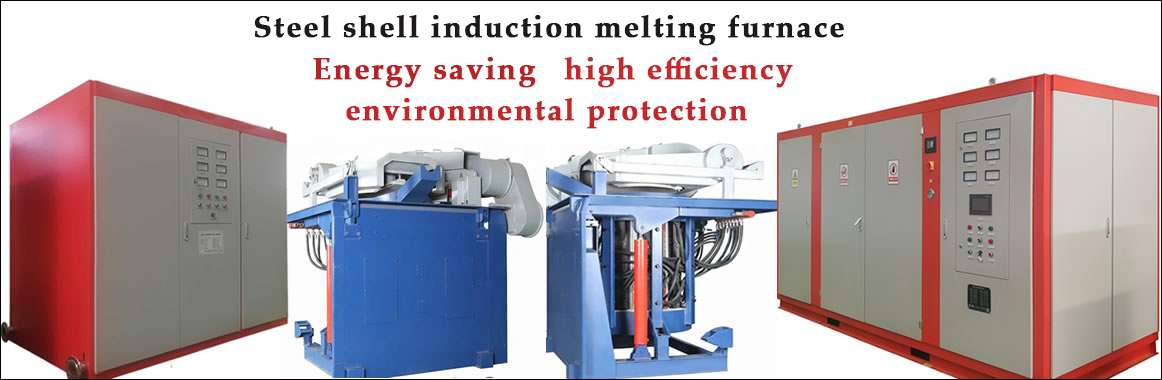Contact
Tel: 18037961302
Sales hot line ( 24 hours  service): 18037961302

E-Mail: firstfurnace@gmail.com

whatsapp:+8618037961302

Adress: Luoxin Industrial Park, Luoyang, Henan
Electric furnace technical information

Basic configuration of steel pipe induction heating equipment

Steel pipe online heating equipment configuration

The steel pipe is heated online before sizing, and the combined through-induction furnace is provided with three combined sections. Each combined section includes an intermediate frequency power supply, a capacitor cabinet and two inductors, and a transport roller path between the inductors. The steel pipe runs at a certain speed. The system is powered by three 12-pulse rectifier transformers. The whole set of equipment is controlled by a computer control system to meet the heating requirements of steel pipes. Three power cooling systems use three feng shui coolers. Attached:

(Steel wire online heating power capacitor water cooling equipment configuration shown in Figure 1-1)

Working pressure is 0.2~0.3Mpa; working flow is 20M3/h

The calculated values are as follows:

It is known that the power supply is 1500KW, the heat generation coefficient per kilowatt of power supply is 0.09, the heat generation coefficient per kilowatt of capacitor is 0.036, the conversion value of function and heat unit is 860 kcal/h, the average temperature difference between inlet and outlet water is 8°C, and the specific heat capacity of water is 1 kcal. / kg ・ ° C × 1000, the density of water is 1000kg / M3.

Calorific value: 1500 × (0.09 + 0.036) = 189 KW

189×860=162540 kcal/h

135450÷8÷1000=20M3/h

Take 1.1 to 1.25 safety factor: 20 × 1.1 = 22M3 / h

The heat taken away by the pump water volume is: 22×8×1000=16700 kcal/h

Conclusion: 176000 kcal / h> 162540 kcal / h, so 1500KW power cooling water 25M3 / h is to meet the equipment cooling.

The sensor cooling water adopts net water: working flow 50T/h, working pressure 0.2～0.3Mpa

It is known that the power supply power is 1500KW, the inductance coefficient per kW of the inductor is 0.3, the function and heat unit conversion value is 860 kcal/h, the temperature difference between the inlet and outlet water is 8°C, and the specific heat capacity of water is 1 kcal/kg・°C×1000. The density is 1 000 kg / M3.

Then the heat generated by the sensor is: 1500×0.3=450KW

450×860=387000 kcal/h

Pump selection: 387000÷8÷1000≈48.4M3/h

Take 1.25 safety factor: 48.4×1.1=53.21M3/h

The heat taken away by the pump is: 53.21×8×1000=425700 kcal/h

Conclusion: 425700 kcal / h> 387000 kcal / h, so 1500KW sensor cooling water 50M3 / h is to meet the equipment cooling requirements.